# Texas Go Math Kindergarten Module 4 Assessment Answer Key

Refer to our Texas Go Math Kindergarten Answer Key Pdf to score good marks in the exams. Test yourself by practicing the problems from Texas Go Math Kindergarten Module 4 Assessment Answer Key.

## Texas Go Math Kindergarten Module 4 Assessment Answer Key

Concepts and Skills

DIRECTIONS: 1. Write the number of objects ¡n each set. (TEKS K.2.B) 2-3. Count and tell how many. Write the number. (TEKS K.2.B)

Question 1.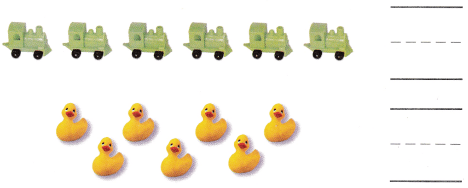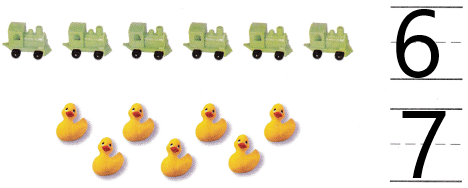Explanation:
There are 6 engines and 7 ducks so, i wrote the numbers 6 and 7.

Question 2.Explanation:
There are 8 counters in the image so, i wrote the number 8.

Question 3.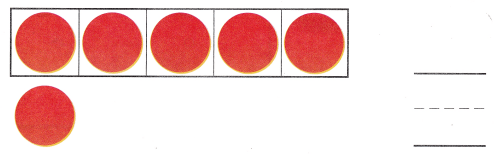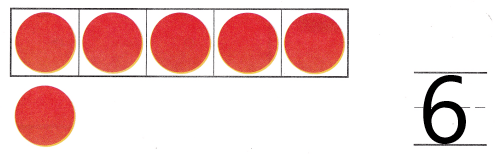Explanation:
There are 6 counters in the image so, i wrote the number 6.

DIRECTIONS: 4. Place counters as shown. Count and tell how many. Write the number, (TEKS K.2.B) 5. How many more than 5 is8? Write the number. (TEKS K.2.B) 6. Mark under the number that shows how many eggs. (TEKS K.2.B)

Question 4.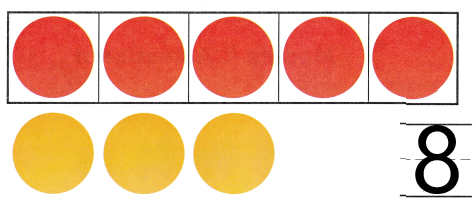Explanation:
There are 5 red counters and 3 yellow counters.So, there are 8 counters in all.I wrote the number 8.

Question 5.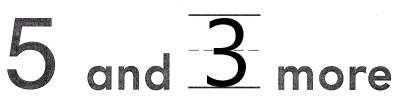Explanation:
3 ore than 5 is 8.So, i wrote the number 3 in the blank.

Question 6.
Texas Test Prep